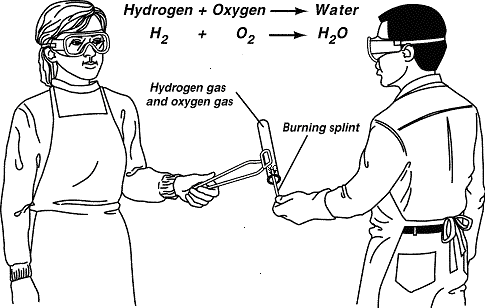Chemical Equations
a year ago
achorn
Save
Edit
Host a game
Live GameLive
Homework
Solo Practice
Practice20 QuestionsShow answers
• Question 1
30 seconds
Q. How many atoms of carbon (C) are in C6H12O6?
3
6
12
24
• Question 2
30 seconds
Q. How many Sodium (Na) are in 6NaCl?
1
12
6
• Question 3
30 seconds
Q. How many Aluminum are in Al2O3?
3
2
5
• Question 4
30 seconds
Q. How many Hydrogen are in 4H2O?
6
8
2
4
• Question 5
120 seconds
Q. Which of the following shows the correct way to balance the chemical equation?
Fe + O2 -> Fe2O3
4Fe + 3O2 -> 2Fe2O3
2 Fe + 3O2 -> Fe2O6
4 Fe + O6 -> 2Fe2O3
None of the options are correctly balanced.
• Question 6
120 seconds
Q. Which of the following reactions is balanced?
2CH4 + O2 -> CO2 + H2O
CH4 + 2O2 -> CO2 + H2O
CH4 + 2O2 -> CO2 + 2H2O
None of the chemical equations are balanced.
• Question 7
60 seconds
Q. How many HCl molecules do you need to balance this equation?
Mg +  __HCl --->  MgCl2 + H2
1
2
3
4
• Question 8
120 seconds
Q. Balance this equation.
_CF+ _Br-- _CBr+ _F2
2,1,2,1
1,2,2,1
1,2,1,2
2,2,2,2
• Question 9
120 seconds
Q. Balance this equation.
_CH4 + _O--> _CO+ _H2O
1,2,1,1
2,1,2,1
1,2,1,2
0,2,0,2
• Question 10
120 seconds
Q. Balance this equation
_Al +_HCl --> _H+_AlCl3
2, 6, 3, 2
4, 12, 3, 4
2, 1, 4, 5
• Question 11
30 seconds
Q. The substances listed on the right side of a chemical equation are the
Yields
Reactants
Products
Precipitates
• Question 12
30 seconds
Q. The substances listed on the left side of a chemical equation are the
Products
Coefficients
Precipitates
Reactants
• Question 13
30 seconds
Q. In chemical reactions, what does the principle of conservation of mass mean?
Matter is not created or destroyed.
The total mass of the reactants is greater than the total mass of the products.
The total mass of the reactants is less than the total mass of the products.
Matter is not changed.
• Question 14
30 secondsQ. Identify the reactant(s) and product(s) of the reaction.
All three are reactants and none are products.
Hydrogen and water are the reactants, and oxygen is the product.
Water and oxygen are the reactants, and hydrogen is the product.
Hydrogen and oxygen are the reactants, and water is the product.
• Question 15
30 seconds
Q.

Which is the Law of Conservation of Matter?

matter cannot be created or destroyed, it only rearranges

matter can be created but it cannot be destroyed, it never rearranges

matter can be destroyed but it cannot be created, it usually just rearranges

matter is an unproven theory created by Hans Geiger

• Question 16
120 seconds
Q. Which one is a compound?
Co
Br
Fe
CO
• Question 17
120 seconds
Q. Which one is an element?
Co
CO
CO2
CO3-2
• Question 18
120 seconds
Q. How many elements are found in the following molecule? Mg(ClO3)2
1
4
3
5
• Question 19
900 seconds
Q. Which number should go in the blank?
_ H2 + O2 -> 2 H2O
1
2
3
0
• Question 20
900 seconds
Q. Which number should go in the blank?
2 Na+ _ Cl2 -> 2 NaCl